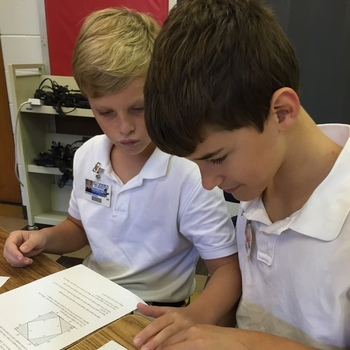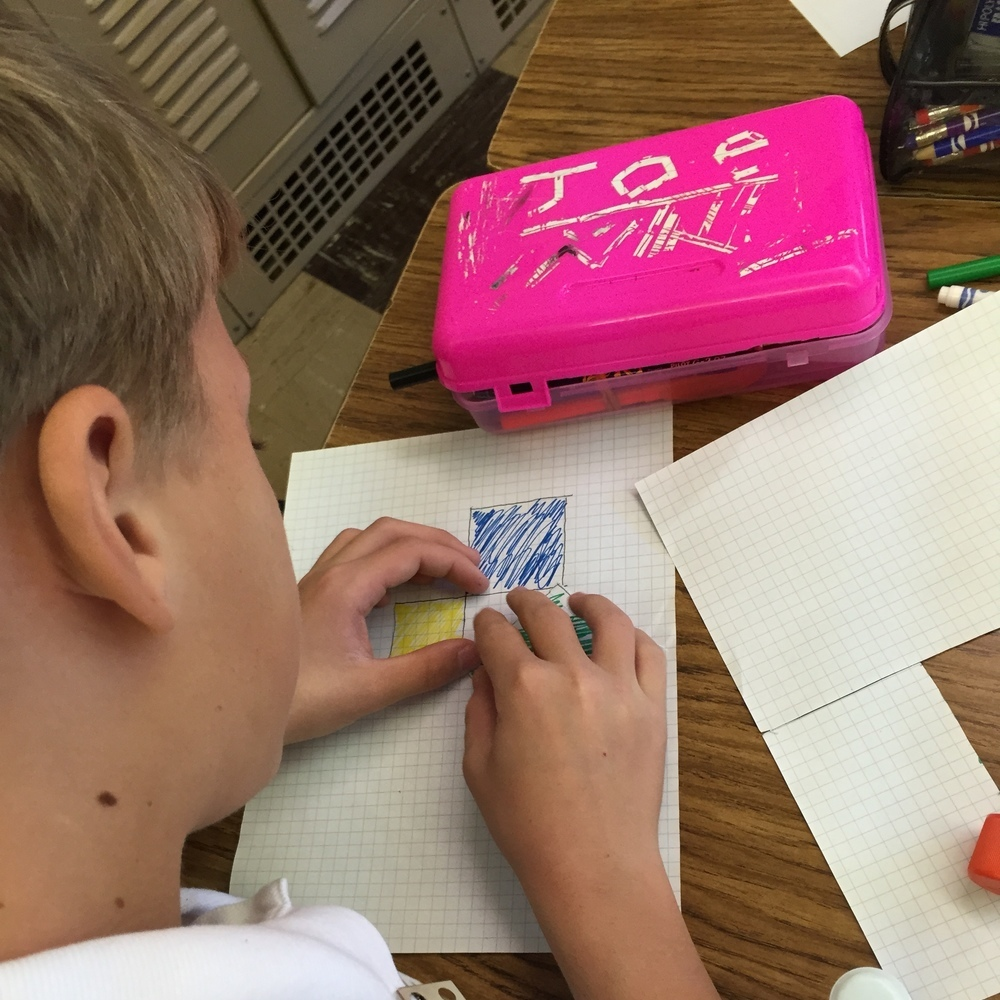# 7th Graders Complete Their First Proofs

•October 5, 2015
There were lots of eurekas in 7th grade accelerated math as students worked in pairs and then two different groups to discover what Pythagoras figured out 25 centuries ago. Working in two different approaches, students constructed right triangles of varying side lengths to determine the relationship between the areas of squares created when using each side of a right triangles as one side of a square. They concluded that the square of the hypotenuse equals the sum of the squares of the two legs: a 2 + b 2 = c 2, otherwise known as the Pythagorean Theorem.